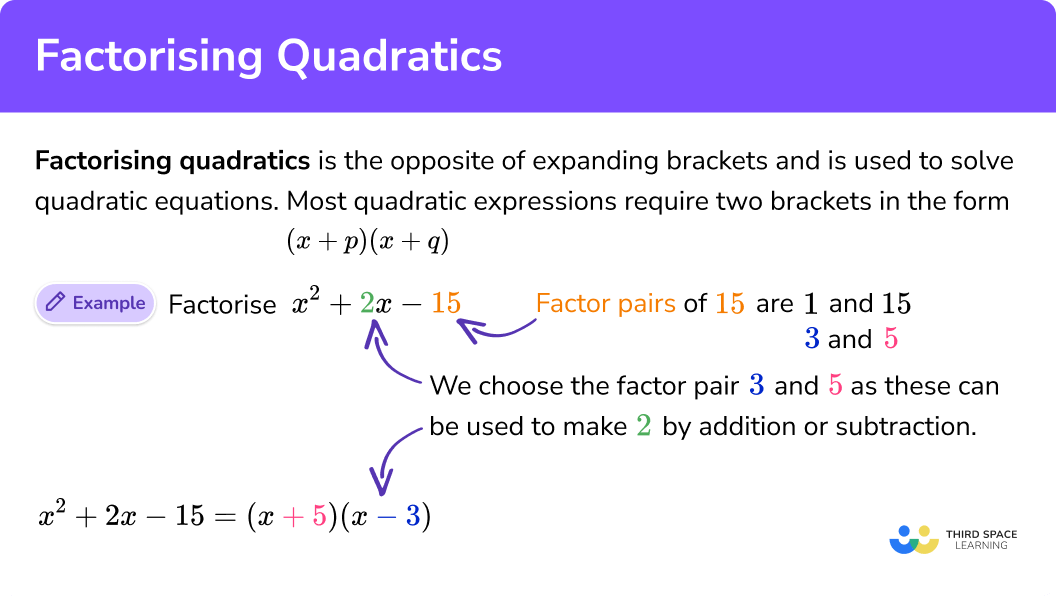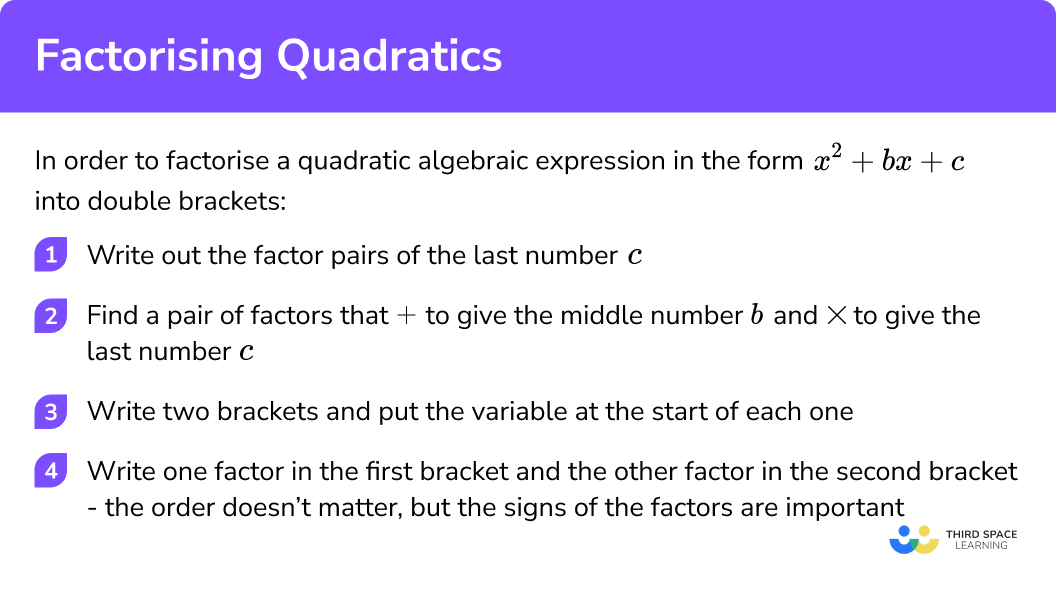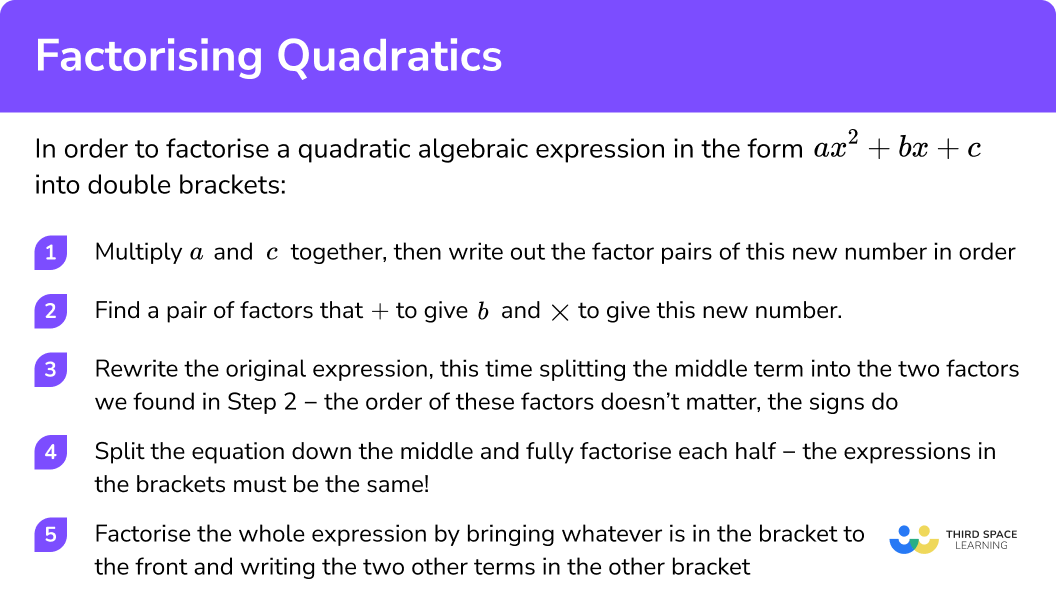Here we will learn about factorising quadratics; we will explore what quadratic expressions are and the steps needed to factorise into double brackets.

There are also factorising quadratics worksheets based on Edexcel, AQA and OCR exam questions, along with further guidance on where to go next if you’re still stuck.

First of all, let’s have a quick recap on quadratic expressions.

## What is a quadratic expression?

A quadratic expression in maths is an expression including a squared term or square number i.e. a term up to x2.

The highest power for a quadratic expression is 2.

The general form of a quadratic expression is:

$\color{#FE47EC}ax^{2}\color{#00BC89}{+b}x\color{#7C4DFF}{+c}$

a is the coefficient (number in front) of the x2 term

b is the coefficient (number in front) of the x term

c is the constant term (number on its own)

e.g.

$x^{2}\color{#00BC89}{-2}x\color{#7C4DFF}{+1}$

$\color{#FE47EC}2x^{2}\color{#00BC89}{+3}x\color{#7C4DFF}{-2}$

We factorise quadratic expressions of this sort using double brackets. There are different methods we can use depending on whether the coefficient of x2 is greater than 1.

Factorising, or factoring quadratic equations is the opposite of expanding brackets and is used to solve quadratic equations.

For example, in the form of x2 + bx + c requires two brackets (x + d) (x + e).

1. Write out the factor pairs of the last number (c).
2. Find a pair of factors that + to give the middle number (b) and multiply to give the last number (c).
3. Write two brackets and put the variable at the start of each one.
4. Write one factor in the first bracket and the other factor in the second bracket. The order isn’t important, but the signs of the factors are.If you’re looking for a summary of all the different ways you can factorise expressions then you may find it helpful to start with our main factorising lesson or look in detail at the other lessons in this section.

A quadratic equation is a quadratic expression that is equal to something. We can solve quadratic equations by using factorisation (or factoring), the quadratic formula or by completing the square.

Step by step guide: Quadratic equations

## Factorising quadratics in the form x2 + bx + c

To factorise a quadratic expression in the form x2 + bx + c we need double brackets. Factorisation into double brackets is the reverse process of expanding double brackets.

In this case, the coefficient (number in front) of the x2 term is 1 (a=1). These are known as monic quadratic.

## How to factorise quadratics: x2 + bx + c (double brackets)

In order to factorise a quadratic algebraic expression in the form x2 + bx + c into double brackets:

1. Write out the factor pairs of the last number (c).
2. Find a pair of factors that + to give the middle number (b) and ✕ to give the last number (c).
3. Write two brackets and put the variable at the start of each one.
4. Write one factor in the first bracket and the other factor in the second bracket. The order isn’t important, the signs of the factors are.

### Explain how to factorise quadratics: x² + bx + c (double brackets)## Factorising quadratics examples: x2 + bx + c (double brackets)

### Example 1: with +x coefficient and a +constant

Fully factorise:

$x^2 \color{#00BC89}{+ 6x}\color{#7C4DFF} {+ 5}$

1. Write out the factor pairs of the last number (5) in order.

$x^2 \color{#00BC89}{+ 6x}\color{#7C4DFF} {+ 5}$

Factors of 5:
1, 5

2We need a pair of factors that + to give the middle number (6) and ✕ to give the last number (5).

$x^2 \color{#00BC89}{+ 6x}\color{#7C4DFF} {+ 5}$

Factors of 5:
1, 5

1 + 5= 6

15= 5

(It’s a good idea to do a quick check that we have the correct numbers)

Remember: to multiply two values together to give a positive answer, the signs must be the same

3Write two brackets and put the variable at the start of each one (x in this case).

$(x\qquad)(x\qquad)$

4Write one factor in the first bracket and the other factor in the second bracket. The order isn’t important, the signs of the factors are.

$(x+\color{#FF9100}1)(x+\color{#FF9100}5)$

We have now fully factorised the quadratic expression.

We can check the answer by multiplying out the brackets!

$(x+1)(x+5)=x^{2}+6x+5$

### Example 2: with -x coefficient and a –constant

Fully factorise:

$x^2 – 2x – 24$

Write out the factor pairs of the last number (24) in order

We need a pair of factors that + to give the middle number (-2) and ✕ to give the last number (-24).

Write two brackets and put the variable at the start of each one (x in this case).

Write one factor in the first bracket and the other factor in the second bracket. The order isn’t important, the signs of the factors are.

### Example 3: with +x coefficient and a –constant

Fully factorise:

x2 + x – 20

Write out the factor pairs of last number (20) in order.

We need a pair of factors that + to give the middle number (1) and ✕ to give the last number (-20).

Write two brackets and put the variable at the start of each one (x in this case)

Write one factor in the first bracket and the other factor in the second bracket. The order isn’t important, the signs of the factors are.

### Example 4: with -x coefficient and a +constant

Fully factorise:

$x^2 – 8x + 15$

Write out the factor pairs of the last number (15) in order.

We need a pair of factors that + to give the middle number (-8) and ✕ to give the last number (15).

Write two brackets and put the variable at the start of each one (x in this case)

Write one factor in the first bracket and the other factor in the second bracket.

## Practice factorising quadratics questions: x2 + bx + c (double brackets)

1. Fully factorise:

x^{2}+5x+6

(x+5)(x+6)(x+3)(x+2)(x+1)(x+6)(x+3)(x+3)We need numbers that have a product of 6 and a sum of 5 . By considering the factors of 6 , we conclude that x^{2}+5x+6=(x+3)(x+2) .

2. Fully factorise:

x^{2}+10x+21

(x+3)(x+7)(x+1)(x+21)(x+10)(x+21)(x+1)(x+10)We need numbers that have a product of 21 and a sum of 10 . By considering the factors of 21 , we conclude that x^{2}+10x+21=(x+3)(x+7) .

3. Fully factorise:

x^{2}-x-12

(x-4)(x+3)(x+4)(x-3)(x-1)(x+12)(x-1)(x-12)We need numbers that have a product of -12 and a sum of -1 . By considering the factors of -12 , we conclude that x^{2}-x-12=(x-4)(x+3) .

4. Fully factorise:

x^{2}+3x-18

(x+2)(x-9)(x+6)(x-3)(x+3)(x-6)(x-6)(x-3)We need numbers that have a product of -18 and a sum of 3 . By considering the factors of -18 , we conclude that x^{2}+3x-18=(x+6)(x-3) .

5. Fully factorise:

x^{2}-6x+8

(x-2)(x-6)(x-2)(x+4)(x+2)(x-4)(x-2)(x-4)We need numbers that have a product of 8 and a sum of -6 . By considering the negative factors of 8 , we conclude that x^{2}-6x+8=(x-2)(x-4) .

6. Fully factorise:

x^{2}-10x+24

(x-4)(x-6)(x-2)(x-12)(x+4)(x-6)(x-4)(x+6)We need numbers that have a product of 24 and a sum of -10 . By considering the negative factors of 24 , we conclude that x^{2}-10x+24=(x-4)(x-6) .

## Factorising quadratics GCSE questions: x2 + bx + c (double brackets)

1. Factorise: x2 + 3x – 10

(x – 2)(x + 5)

(2 marks)

2. Factorise: y2 – 10y + 16

(y – 2)(y – 8)

(2 marks)

3. Factorise: x2 – 12x + 27

(x – 3)(x – 9)

(2 marks)

## Factorising quadratics in the form ax2 + bx + c

To factorise a quadratic expression in the form ax2 + bx + c we need double brackets. Factorising into double brackets is the reverse process of expanding double brackets.

In this case the coefficient (number in front) of the x2 term is greater than 1 (a > 1). These are known as non-monic quadratics.

## How to factorise quadratics: ax2 + bx + c (double brackets)

In order to factorise a quadratic algebraic expression in the form ax2 + bx + c into double brackets:

1. Multiply the end numbers together (a and c) then write out the factor pairs of this new number in order.
2. We need a pair of factors that + to give the middle number (b) and ✕ to give this new number.
3. Rewrite the original expression, this time splitting the middle term into the two factors we found in step 2. The order of these factors doesn’t matter, the signs do.
4. Split the equation down the middle and fully factorise each half. The expressions in the brackets must be the same!
5. Factorise the whole expression by bringing the contents of the bracket to the front and writing the two other terms in the other bracket.

### Explain how to factorise quadratics: ax² + bx + c (double brackets)## Factorising quadratics examples: ax2 + bx + c (double brackets)

### Example 1: with +x coefficient and a + constant

Fully factorise:

$2x^2 + 5x + 3$

1. Multiply the end numbers together (2 and 3) then write out the factor pairs of this new number in order.

2x2 + 5x + 3

2 × 3 = 6

Factors of 6:
1, 6
2, 3

2We need a pair of factors that + to give the middle number (5) and ✕ to give this new number (6).

2x2 + 5x + 3

2 × 3 = 6

Factors of 6:
1, 6
2, 3

+ 5
✕ 6

2 + 3 = 5 ✔
2 x 3 = 6 ✔

Remember: to x two values together to give a positive answer, the signs must be the same.

3Go back to the original equation and rewrite it this time splitting the middle term into the two factors we found in step 2 – the order of these factors doesn’t matter, the signs do.

2x2 + 5x + 3

2x2 + 2x + 3x + 3

4Split the equation down the middle into two halves and fully factorise each half – the expressions in the brackets must be the same!

2x2 + 5x + 3

2x2 + 2x + 3x + 3

2x(x + 1) + 3(x + 1)

2x(x + 1) + 3(x + 1)

5Now factorise the whole expression by bringing whatever is in the bracket to the front and writing the two other terms in the other bracket.

(x + 1) ( 2x \; + \; 3 )

The order of the brackets doesn’t matter

We have now fully factorised the quadratic expression.

We can check the answer by multiplying out the brackets!

(x + 1)(2x + 3) = 2x2 + 5x + 3

### Example 2: with +x coefficient and a –constant

Fully factorise:

$2x^2 + 3x – 2$

Multiply the the end numbers together (2 and -2) then write out the factor pairs of this new number in order.

We need a pair of factors that + to give the middle number (3) and ✕ to give this new number (-4)

Go back to the original equation and rewrite it this time splitting the middle term into the two factors we found in step 2 – the order of these factors doesn’t matter, the signs do.

Split the equation down the middle into two halves and fully factorise each half – the expressions in the brackets must be the same!

Now factorise the whole expression by bringing whatever is in the bracket to the front and writing the two other terms in the other bracket.

### Example 3: with -x coefficient and a –constant

Fully factorise:

$3x^2 – 2x – 8$

Multiply the the end numbers together (3 and -8) then write out the factor pairs of this new number in order.

We need a pair of factors that + to give the middle number (-2) and to ✕ give this new number (-24)

Go back to the original equation and rewrite it this time splitting the middle term into the two factors we found in step 2 – the order of these factors doesn’t matter, the signs do.

Split the equation down the middle into two halves and fully factorise each half – the expressions in the brackets must be the same!

Now factorise the whole expression by bringing whatever is in the bracket to the front and writing the two other terms in the other bracket.

### Example 4: with -x coefficient and a +constant

Fully factorise:

$6x^2 – 7x + 2$

Multiply the the end numbers together (6 and 2) then write out the factor pairs of this new number in order.

We need a pair of factors that + to give the middle number (-7) and ✕ to give this new number (12)

Go back to the original equation and rewrite it this time splitting the middle term into the two factors we found in step 2 – the order of these factors doesn’t matter, the signs do.

Split the equation down the middle into two halves and fully factorise each half – the expressions in the brackets must be the same!

Now factorise the whole expression by bringing whatever is in the bracket to the front and writing the two other terms in the other bracket.

## Practice factorising quadratics questions: ax2 + bx + c (double brackets)

1. Fully factorise:

2x^{2}+5x+2

(x+1)(2x+2)(2x+1)(x+2)(2x+2)(x+2)(2x+5)(x+2)Using the method from the above lesson, we can rewrite 2x^{2}+5x+2 as 2x^{2}+4x+x+2 which can be factorised as 2x(x+2)+1(x+2) or more concisely, (2x+1)(x+2) .

2. Fully factorise:

2x^{2}+x-6

(2x-3)(x+2)(2x+3)(x+2)(2x-3)(x-2)(2x-1)(x+6)Using the method from the above lesson, we can rewrite 2x^{2}+x-6 as 2x^{2}-3x+4x-6 which can be factorised as x(2x-3)+2(2x-3) or more concisely, (2x-3)(x+2) .

3. Fully factorise:

2x^{2}-14x+20

(2x-4)(x-5)2(x-2)(x-5)(2x-1)(x-20)(x-4)(2x-5)For the given expression, 2x^{2}-14x+20 first factor out the HCF of 2 , giving 2[x^{2}-7x+10] then use the method from the lesson to factorise this simpler expression, hence 2[x^{2}-5x-2x+10] or 2[x(x-5)-2(x-5)] so that the fully factorised expression is 2(x-2)(x-5) .

4. Fully factorise:

3x^{2}-7x-6

(3x+3)(x-2)(3x-2)(x+3)(3x+2)(x-3)(x+2)(3x-3)Using the method from the above lesson, we can rewrite 3x^{2}-7x-6 as 3x^{2}-9x+2x-6 which can be factorised as 3x(x-3)+2(x-3) or more concisely, (3x+2)(x-3) .

5. Fully factorise:

3x^{2}-7x+2

(3x+1)(x-2)(3x-1)(x+2)(x-1)(3x-2)(3x-1)(x-2)Using the method from the above lesson, we can rewrite 3x^{2}-7x+2 as 3x^{2}-6x-x+2 which can be factorised as 3x(x-2)-(x-2) or more concisely, (3x-1)(x-2) .

6. Fully factorise:

4x^{2}-18x+8

(4x+2)(x-4)(4x-2)(x+4)2(2x-1)(x-4)(4x-2)(x-4)For the given expression, 4x^{2}-18x+8 first factor out the HCF of 2 , giving  2(2x^{2}-9x+4) then use the method from the lesson to factorise this simpler expression, hence 2[2x^{2}-8x-x+4] or 2[2x(x-4)-(x-4)] so that the fully factorised expression is 2(2x-1)(x-4) .

## Factorising quadratics GCSE questions: ax2 + bx + c (double brackets)

1. Factorise: 2x2 + 9x + 4

(2x + 1)(x + 4)

(2 marks)

2. Factorise: 2y2 – y – 3

(2y – 3)(y + 1)

(2 marks)

3. Factorise: 2x2 – x – 10

(2x – 5)(x + 2)

(2 marks)

## Common misconceptions

• The order of the brackets

When we multiply two values the order doesn’t matter. This is true for the brackets when factorising quadratics

e.g. 2 × 3 = 3 × 2

It is exactly the same here.

(x – 6)(x + 4) means (x – 6)(x + 4)

So,

(x – 6)(x + 4)=(x + 4)(x – 6)

• The signs of the factors

It is common for students to get confused regarding the signs of the factors in the brackets, especially with negative factors.

e.g. if the factors are -6 and 4, the numbers is the brackets must be:
(x – 6)(x + 4)

• Multiplying two numbers to give a +

For two numbers to multiply to give a + their signs must be the same, that is a negative x a negative or a positive x a positive.

+ × + = +

e.g 2 × 3 = 6

4 × 5=20

– × – = +

e.g -2 × -3 = 6

-4 × -5= 20

• Multiplying two numbers to give a

For two numbers to multiply to give a – their signs must be different, that is a negative x a positive or a positive x a negative.

+ × – = –

e.g 2 × -3= -6

4 × -5= -20

– × + = –

e.g -2 × 3= -6

-4 × 5= -20

• The signs are important for the end numbers as well

e.g. 3x^{2} - 2x - 8

3 x -8 = -24 NOT 24

## Learning checklist

You have now learnt how to:

• Manipulate algebraic expressions by taking out common factors to factorise into a single bracket
• Factorise quadratic expressions of the form x^{2} + bx + c
• Factorise quadratic expressions of the form of the difference of two squares
• Factorising quadratic expressions of the form ax^{2} + bx + c (H)

## Still stuck?

Prepare your KS4 students for maths GCSEs success with Third Space Learning. Weekly online one to one GCSE maths revision lessons delivered by expert maths tutors.

Find out more about our GCSE maths tuition programme.